# Orthogonalization

orthogonalization process

An algorithm to construct for a given linear independent system of vectors in a Euclidean or Hermitian spacean orthogonal system of non-zero vectors generating the same subspace in. The most well-known is the Schmidt (or Gram–Schmidt) orthogonalization process, in which from a linear independent system, an orthogonal system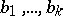is constructed such that every vector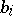(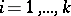) is linearly expressed in terms of, i.e.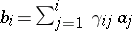, where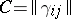is an upper-triangular matrix. It is possible to construct the systemsuch that it is orthonormal and such that the diagonal entries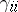ofare positive; the systemand the matrixare defined uniquely by these conditions.

The Gram–Schmidt process is as follows. Put; if the vectors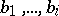have already been constructed, then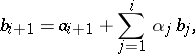where

 αj = - (ai+1 , bj) / (bj , bj),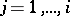, are obtained from the condition of orthogonality of the vector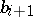to. The geometric sense of this process comprises the fact that at every step, the vectoris perpendicular to the linear hull of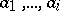drawn to the end of the vector. The product of the lengths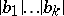is equal to the volume of the parallelepiped constructed on the vectors of the system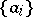as edges. By normalizing the vectors, the required orthonormal system is obtained. An explicit expression of the vectorsin terms of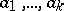is given by the formula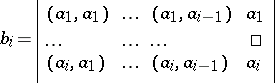/Gi-1

where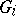is the Gram determinant of the system, with G0=1 by definition. (The determinant at the right-hand side has to be formally expanded by the last column).

The norm of these orthogonal vectors is given by ||bi||=SQRT(Gi/Gi-1). Thus, the corresponding orthonormal system takes the form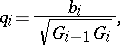· Gi-1 = bi · SQRT(Gi-1 / Gi)

This process can also be used for a countable system of vectors.

The Gram–Schmidt process can be interpreted as expansion of a non-singular square matrix in the product of an orthogonal (or unitary, in the case of a Hermitian space) and an upper-triangular matrix with positive diagonal entries, this product being a particular example of an Iwasawa decomposition.